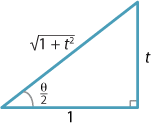### The $$t$$ formulas

Introducing the parameter $$t = \tan\dfrac{\theta}{2}$$ turns out to be a very useful tool in solving certain types of trigonometric equations and also in finding certain integrals involving trigonometric functions. The basic idea is to relate $$\sin\theta$$, $$\cos\theta$$ and even $$\tan\theta$$ to the tangent of half the angle. This can be done using the double angle formulas.

We let $$t= \tan\dfrac{\theta}{2}$$ and so we can draw the following triangle with sides 1, $$t$$, $$\sqrt{1+t^2}$$.Detailed description of diagram

Now $$\sin 2\alpha = 2\sin\alpha\cos\alpha$$, so replacing $$2\alpha$$ with $$\theta$$ we have

$\sin \theta = 2 \sin\dfrac{\theta}{2}\, \cos\dfrac{\theta}{2}.$

From the triangle, we have

$\sin \theta = 2 \times \dfrac{t}{\sqrt{1+t^2}} \times \dfrac{1}{\sqrt{1+t^2}} = \dfrac{2t}{1+t^2}.$

This is referred to as the $$t$$ formula for $$\sin \theta$$.

Exercise 20

Use the geometric method above to derive the $$t$$ formula

$\cos \theta = \dfrac{1-t^2}{1+t^2}$ and deduce that $$\tan\theta = \dfrac{2t}{1-t^2}$$, for $$t \ne \pm 1$$.

Exercise 21

The geometric proof of the $$t$$ formula for $$\sin\theta$$ given above assumes that the angle $$\dfrac{\theta}{2}$$ is acute. Give a general algebraic proof of the formula.

One application of the $$t$$ formulas is to solving certain types of trigonometric equations.

#### Example

Solve $$\cos\theta + \sin\theta = \dfrac{1}{2}$$, for $$0^\circ \leq \theta \leq 360^\circ$$, correct to one decimal place.

#### Solution

Put $$t = \tan\dfrac{\theta}{2}$$. Then

$\dfrac{1-t^2}{1+t^2} + \dfrac{2t}{1+t^2} = \dfrac{1}{2}.$

This rearranges to $$3t^2-4t-1=0$$, whose solutions are $$t = \dfrac{2\pm\sqrt{7}}{3} \approx 1.549, -0.215$$. Taking the inverse tangents (in degrees) and doubling, we obtain the solutions $$\theta \approx 114.3^\circ, 335.7^\circ$$ in the given range.

Note. Some care is required when using the $$t$$ formulas to find solutions of an equation, as there may be a solution $$\theta$$ for which $$\tan {\dfrac\theta2}$$ is undefined.

Next page - History